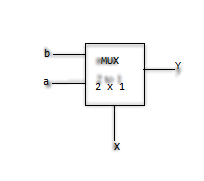# GATE | GATE-CS-2014-(Set-3) | Question 65

Consider the following combinational function block involving four Boolean variables x, y, a, b where x, a, b are inputs and y is the output.

 `f (x, y, a, b) ` `{ ` `   ``if` `(x is 1) y = a; ` `   ``else` `y = b; ` `} `

Which one of the following digital logic blocks is the most suitable for implementing this function?
(B) Priority encoder
(C) Multiplexer
(D) Flip-flop

Explanation: This function can be interpreted as having two inputs a, b and select signal x. Output y will depend on the select signal x.

Function will be like (ax+bx’)

Its implementation will be likeSo ans is ( C) part.

Quiz of this Question

My Personal Notes arrow_drop_up
Article Tags :

Be the First to upvote.

Please write to us at contribute@geeksforgeeks.org to report any issue with the above content.